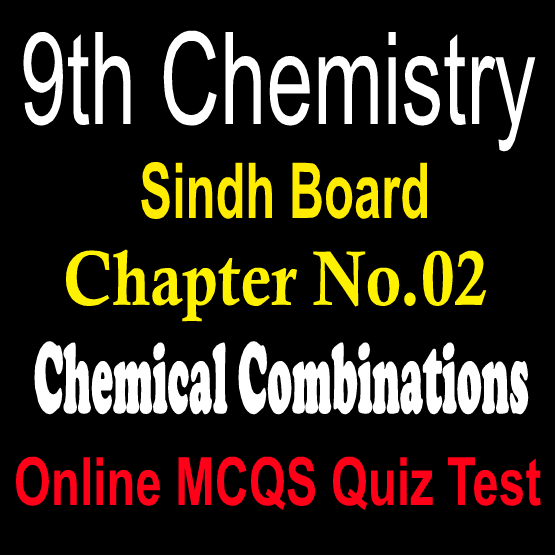# 9th Chemistry Unit 2 Chemical Combinations MCQs Sindh Board

9th Grade Chemistry Chapter 2 Chemical Combinations Sindh Board MCQs Notes Online Chapter Wise Question Answers. Sindh Boards Chemistry Subject Notes For Exams Preparations Karachi, Jamshoro, Sindh Boards. Chemistry class Ninth sindh board notes Unit 2 Chemical Combinations Most Repeated Multiple Choice Questions With Answers. Chemistry notes for class IX sindh board karachi Boards.9th Chemistry Sindh Board MCQs

Q.1: Mass can neither be created nor destroyed during a chemical reaction, is the law of?
1. reciprocal proportion
2. conservation of mass
3. constant composition
4. multiple proportion
B
Q.2: Law of Multiple proportion was proposed by?
1. Ritcher
2. Lavoisier
3. Louise Proust
4. John Dalton
D
Q.3: Berzelius performed the experiments on the preparation of?
1. ZnS
2. PbS
3. FeS
4. H2S
B
Q.4: A combination of symbols of atoms or ions, that are held together chemically in a compound is called?
1. simplest formula
2. molecular formula
3. empirical formula
4. formula
D
Q.5: 2 moles of CaCO3 is equal to?
1. 160g
2. 200g
3. 88g
4. 100g
B
Q.6: 23 a.m.u. of Na is equal to?
1. 2 moles
2. 4 moles
3. 3 moles
4. 1 mole
D
Q.7: CaCO3 CaO + CO2 is an example of:?
1. decomposition reaction
2. combustion reaction
3. single displacement
A
Q.8: A reaction in which substances reacts with oxygen is?
1. combustion reaction
2. single displacement reaction
4. decomposition reaction
A
Q.9: Which type of reactions are neutralization and hydrolysis reaction?
1. single displacement
3. decomposition
4. double displacement
D
Q.10: The number in front of the formula in a chemical equation is called?
1. subscript
2. Co efficient
3. expression
4. none of these
B
Q.11: Symbol represents?
1. light
2. state of matter
3. heat
4. catalyst
C
Q.12: How many grams atoms arc therein 5 moles of carbons?
1. 24g
2. 36g
3. 60g
4. 12g
C
Q.13: NA represents?
1. mole
2. mass number
3. atomic number
D
Q.14: A reaction in which two or more substances react to form a single substance is called?
1. combustion reaction
2. displacement reaction
3. decomposition reaction
D
Q.15: Avogadros Number shows the number of?
1. electrons
2. particles
3. neutrons
4. protons
B
Q.16: The formulas for all atoms in an empirical formula of a compound is?
1. Empirical formulas
2. Structural formulas
3. Chemical formulas
4. Molecular formulas
A
Q.17: In 1961, the standard for atomic masses was designated by the isotope of:?
1. C\-12
2. C-13
3. H-1
4. C-14
A
Q.18: One mole of any substance contains?
1. Unequal number of particles
2. Equal number of particles
4. Dalton numbers of particles
C
Q.19: Avogadro number is constant. Its value is:?
1. 3.02 x 1021
2. 3.02 x 1022
3. 3.02 x 1023
4. 3.02 x 1024
C
Q.20: Benzene has molecular formula C6H6. Its empirical formula will be?
1. C18H18
2. C2H2
3. C12H12
4. CH
D
Q.21: One mole of CO2 contains CO2?
1. 22g
2. 12g
3. 32g
4. 44g
D
Q.22: In water, the ratio between the masses of hydrogen and oxygen is:?
1. 1:2
2. 1:8
3. 2:1
4. 2:16
B
Q.23: The mass of one mole of substance is called:?
1. Molecular mass
2. Formula mass
3. Molar mass
4. Atomic mass
C
Q.24: In a chemical equation, reactants are written on the left hand side and products are written on the:?
1. Left
2. Middle
3. Right
4. Upper
C
Q.25: The symbol represents a?
1. No way reaction
2. Half way reaction
3. One way reaction
4. Two way reversible reaction
D
Previous Post
Next Post